# Analytic geometry + vector - math problems

#### Number of problems found: 38

• CoG center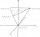Find the position of the center of gravity of a system of four mass points having masses, m1, m2 = 2 m1, m3 = 3 m1, and m4 = 4 m1, if they lie at the vertices of an isosceles tetrahedron. (in all cases, between adjacent material points, the distance
• Perpendicular projectionDetermine the distance of a point B[1, -3] from the perpendicular projection of a point A[3, -2] on a straight line 2 x + y + 1 = 0.
• Place vectorPlace the vector AB, if A (3, -1), B (5,3) in the point C (1,3) so that AB = CO
• Ascend vs. descentWhich function is growing? a) y = 2-x b) y = 20 c) y = (x + 2). (-5) d) y = x-2
• Three points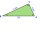Three points K (-3; 2), L (-1; 4), M (3, -4) are given. Find out: (a) whether the triangle KLM is right b) calculate the length of the line to the k side c) write the coordinates of the vector LM d) write the directional form of the KM side e) write the d
• Vector v4Find the vector v4 perpendicular to vectors v1 = (1, 1, 1, -1), v2 = (1, 1, -1, 1) and v3 = (0, 0, 1, 1)
• Calculate 6Calculate the distance of a point A[0, 2] from a line passing through points B[9, 5] and C[1, -1].
• Vector perpendicularFind the vector a = (2, y, z) so that a⊥ b and a ⊥ c where b = (-1, 4, 2) and c = (3, -3, -1)
• Vector equationLet’s v = (1, 2, 1), u = (0, -1, 3) and w = (1, 0, 7) . Solve the vector equation c1 v + c2 u + c3 w = 0 for variables c1 c2, c3 and decide weather v, u and w are linear dependent or independent
• Angle of the body diagonalsUsing vector dot product calculate the angle of the body diagonals of the cube.
• Coordinates of a centroindLet’s A = [3, 2, 0], B = [1, -2, 4] and C = [1, 1, 1] be 3 points in space. Calculate the coordinates of the centroid of △ABC (the intersection of the medians).
• Parametric formCalculate the distance of point A [2,1] from the line p: X = -1 + 3 t Y = 5-4 t Line p has a parametric form of the line equation. ..
• Find the 10Find the value of t if 2tx+5y-6=0 and 5x-4y+8=0 are perpendicular, parallel, what angle does each of the lines make with the x-axis, find the angle between the lines?
• Coordinates of square verticesThe ABCD square has the center S [−3, −2] and the vertex A [1, −3]. Find the coordinates of the other vertices of the square.
• Find the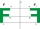Find the image A´ of point A [1,2] in axial symmetry with the axis p: x = -1 + 3t, y = -2 + t (t = are real number)
• Coordinates of vector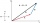Determine the coordinate of a vector u=CD if C(19;-7) and D(-16;-5)
• Find the 5Find the equation of the circle with center at (1,20), which touches the line 8x+5y-19=0
• Two peopleTwo straight lines cross at right angles. Two people start simultaneously at the point of intersection. John walking at the rate of 4 kph in one road, Jenelyn walking at the rate of 8 kph on the other road. How long will it take for them to be 20√5 km apa
• Points collinear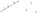Show that the point A(-1,3), B(3,2), C(11,0) are col-linear.
• Three points 2The three points A(3, 8), B(6, 2) and C(10, 2). The point D is such that the line DA is perpendicular to AB, and DC is parallel to AB. Calculate the coordinates of D.

Do you have an exciting math question or word problem that you can't solve? Ask a question or post a math problem, and we can try to solve it.

We will send a solution to your e-mail address. Solved examples are also published here. Please enter the e-mail correctly and check whether you don't have a full mailbox.

Line slope calculator is helpful for basic calculations in analytic geometry. The coordinates of two points in the plane calculate slope, normal and parametric line equation(s), slope, directional angle, direction vector, the length of the segment, intersections of the coordinate axes, etc. Our vector sum calculator can add two vectors given by their magnitudes and by included angle. Analytic geometry - math problems. Vector - math problems.